## Sunday, March 30, 2014

### Gravitational Waves 2

This is the second part of a two-part post on gravitational waves. For the first part, see here.

The previous post introduces gravitational waves, and discusses early attempts at their detection, such as LIGO. Despite LIGO's failure to detect these waves, new instruments promise to increase precision, allowing us to find weaker gravitational waves, and other indirect methods have yielded results. Overall, these techniques will give us new methods of observing our Universe.

LIGO, as well as other early laser interferometer observatories such as VIRGO (a similar detector in Italy), are sometimes known as the "first generation" of gravitational wave detectors. During LIGO's operation, upgrades led to modest increases in sensitivity. However, after the temporary cessation of operations in 2010, more major upgrades were made to LIGO including heavier mirrors and more powerful lasers, which will increase sensitivity and reduce background noise caused by thermal energy. The new Advanced LIGO began operation in 2016, and should have a range of hundreds of millions of light years, ten times that of the original design (see diagram below). These upgraded observatories were the "second generation" of detectors.

The original LIGO could detect gravitational wave sources only within our Local supercluster and its neighbors (small gray sphere), but Advanced LIGO was able to scour an volume of space 1000 times as large for gravitational wave signals (the entire scope of the figure above).

The above diagram shows the actual and estimated sensitivities for different gravitational wave detectors, including LIGO, VIRGO, and their respective upgrades. The x-axis of the graph is the frequency of the gravitational wave (gravitational waves have different frequencies in the same way that electromagnetic waves do) and the y-axis indicates the intensity of the waves. The detectors exhibit different sensitivities to different frequencies; curves that dip lower indicate better detectors. The Einstein GW Telescope is a proposed "third-generation" laser interferometer concept, still in design phase. This design would have the facility be underground to reduce seismic noise and cryogenically cooled to prevent thermal vibrations from altering the distance between mirrors.

Another "third-generation" design concept which would theoretically yield numerous detections is the Laser Interferometer Space Antenna (LISA), a spaced-based model.

LISA would consist of three separate spacecraft, which would create a equilateral triangle of side length 5 million kilometers (3.1 million miles). The above diagram is an artist's conception. This triangle would trail the Earth in heliocentric orbit, and would be very sensitive to different frequencies of gravitational waves than ground-based detectors like LIGO.

The above figure shows that LISA would detect much longer wavelengths than Advanced LIGO (due to LISA's enormous arms). Advanced LIGO could only discover very high frequency oscillations, such as neutron stars rotating very close to each other just before colliding. Such systems are rare and short-lived, since neutron star systems contract and ultimately collide. However, LISA could detect more slowly orbiting binary systems, long before their final collision. These are very common, and many are already known through other means of observation, guaranteeing that LISA would find many sources if it functions correctly.

There are unfortunately no definite plans for launching LISA, but a small test mission, known as LISA Pathfinder, launched in 2015. This small probe contained a tiny interferometer meant to test the LISA concept in space and evaluate the proposal's feasibility.

Ultimately, the most important goal of gravitational-wave observatories is to peer farther into the early universe than could be possible with telescopes measuring electromagnetic radiation. Using ordinary visual telescopes (of sufficient power), we can view objects billions of light years away (seeing them as they were billions of years ago, since it takes light a year to travel each light-year). However, there is a fundamental limit to how far these telescopes can see. Before 380,000 years after the Big Bang (or about 13.8 billion years ago), the temperature of the Universe was too high for electrons to combine with atomic nuclei into atoms, and, since electrons scatter electromagnetic radiation, the Universe was opaque. Thus the "oldest" light in the Universe is from 380,000 years after the Big Bang; it is called the Cosmic Microwave Background (CMB), and traditional telescopes cannot see farther. However, gravitational wave astronomy has the potential to receive signals from earlier periods and study them directly, leading to a greater understanding of the Big Bang.

For an update on recent developments in the detection of gravitational waves, see here!

Sources: https://www.advancedligo.mit.edu/summary.html, http://www.ligo.caltech.edu/docs/G/G080303-00.pdf, http://www.et-gw.eu/, http://www.physik.hu-berlin.de/qom/research/freqref/lisa, http://lisa.nasa.gov/, http://cosmology.berkeley.edu/~yuki/CMBpol/CMBpol.htm, http://www.theguardian.com/science/2014/mar/17/primordial-gravitational-wave-discovery-physics-bicep, http://www.nytimes.com/2014/03/25/science/space/ripples-from-the-big-bang.html?_r=0, "An Ear to the Big Bang" from The Scientific American October 2013 issue

## Saturday, March 22, 2014

### Gravitational Waves 1

Gravitational waves, in brief, are the propagations of gravitational fields through space. Before dealing with gravitational waves directly, we attempt to provide historical context and a way to visualize how the waves work.

In 1865, James Clerk Maxwell (1831-1879) published a paper outlining his theory of electromagnetism, compiling and uniting earlier work into a single theory explaining the properties of both electricity and magnetism. For example, it deals with the properties objects possessing positive and negative charges, and the forces they exert on their environments (electric and magnetic fields). This theory also describes electromagnetic waves, or propagating changes in the electromagnetic field. Such waves are characterized by their wavelength and amplitude.

The above simplified diagram of a wave shows its wavelength and amplitude. We also define the frequency of a wave as the number of oscillations per second. In the diagram above, the wave has a frequency of 2 Hz. For electromagnetic waves, amplitude corresponds to intensity of the wave, and wavelength to type (or in the case of visual light, color). The continuous interval of electromagnetic wavelengths is known as the electromagnetic spectrum and includes many familiar types of radiation, including radio waves, microwaves, infrared rays, visible light, ultraviolet rays, X-rays, and gamma rays (all of these types are discussed in the link above).

Such waves are produced when charged objects move through space, causing a change in electric field. When a charge has moved, it will not exert the same forces on its surroundings as it had previously. The change in field is "carried" by waves, which move at the speed of light, a finite (though very fast) speed. The diagram below shows an example of electromagnetic wave production by a dipole, or a pair of equal and opposite charges.

As the charges oscillate up and down, an electromagnetic wave is produced, and propagates away from the dipole (the blue and red parts of the oscillation are the electric and magnetic field components, respectively). Since the oscillation is periodic, the wave signal it produces is also periodic. The magnitude of the charges forming the dipole determines the amplitude of the generated wave. Also, the frequency of the oscillation determines the frequency of the electromagnetic waves.

At the beginning 20th century, though Maxwell's theory had supplanted earlier understandings of electromagnetism, Newton's was still the dominant paradigm for gravitation. The theories did have similarities, among them the fact that both electromagnetic and gravitational forces shrank with distance in inverse proportion with the square of this distance (F ~ 1/r2). However, while there were both attractive and repulsive electromagnetic forces, gravity was always an attractive force. Another crucial difference was that, as shown above, electromagnetic fields move at the speed of light. Newton's theory, though, simply assumed that all bodies pulled instantaneously on one another. Albert Einstein (1879-1955) developed the theory of general relativity in 1916 and resolved this difference. His theory predicted that differences in gravitational fields would move analogously to electromagnetic fields: using gravitational waves. Further, these postulated gravitational waves would travel at the speed of light.

Gravitational waves, in Einstein's theory, would also be produced in an analogous manner to electromagnetic waves. Instead of oscillating charges, oscillating masses would produce the waves. For example, two massive bodies (such as black holes) orbiting one another at close range would produce gravitational radiation, as in the diagram below.

The conception above illustrates how gravitational waves move away from the orbiting system in all directions. Unlike their electromagnetic counterparts, gravitational waves travel undisturbed through matter, and as a consequence are much more difficult to detect. Nevertheless, they do have a subtle effect on the matter which they pass through. The medium through which gravitational waves travel is the fabric of space itself. The diagram above illustrates distortions of a two-dimensional space fabric; in reality, gravitational waves would cause small "ripples" in our three-dimensional space.

Beginning in the 1960's scientists on Earth have constructed increasingly sophisticated gravitational wave detectors. The first variety were known as Weber bars, or large bars of metal which, if sufficiently isolated from the surrounding environment, could oscllate as gravitational waves passed through them. However, the waves had to be very strong to be detected, and the original models were not up to the task. More modern Weber bars have been supercooled to temperatures very near absolute zero to reduce outside vibrations and increase their sensitivity.

Another method for identifying incoming gravitational waves is known as laser interferometry.

Laser interferometry works by using light beams to measure distances. In the usual design (diagram above), a laser creates a beam of light which is split by a beam-splitting mirror into two beams which travel down the two perpendicular arms of the interferometer. On the return trip, if the arms are exactly the same length, the beams interfere with one another in such a way that all the light travels back to the laser. If, however, the arms have slightly different lengths, some light will be reflected by the beam-splitter into a detector.

The Laser Interferometer Gravitational-Wave Observatory (LIGO) wass one project making use of a laser interferometer to detect gravitational waves. In each of LIGO's facilities (there is one in Louisiana and one in Washington) there was a laser inteferometer with arms four kilometers (2.5 miles) long. Theory held that when a gravitational wave passes through the detector, it distorts space and actually alters the lengths of the arms slightly. Since the arms are perpendicular, the distortions are different, and sufficiently strong waves should then cause the laser beams to be enough out of sync to send light to the detector. There were two LIGO stations to weed out false data and to determine which way gravitational waves moved through the Earth. Despite the precision of LIGO, it did not make any unambiguous detections during its operation (2002-2010).

Despite these setbacks, concepts for more precise instruments and new detectors have since been developed, and the road to gravitational wave detection has also proceeded through more indirect means (see the next post).

Sources: http://www.britannica.com/EBchecked/topic/242499/gravity-wave, http://rsta.royalsocietypublishing.org/content/366/1871/1849.full, http://www.tapir.caltech.edu/~teviet/Waves/differences.html, http://www.geo.mtu.edu/~scarn/teaching/GE4250/EM_wave_lecture.pdf, http://ned.ipac.caltech.edu/level5/ESSAYS/Boughn/figure1.gif, http://upload.wikimedia.org/wikipedia/commons/3/35/Onde_electromagnetique.svg, http://www.vias.org/wirelessnetw/img/wndw-print_img_3.png, spaceplace.nasa.gov, http://en.wikipedia.org/wiki/Gravitational-wave_detector, http://www.learner.org/courses/physics/visual/visual.html?shortname=ligo_interfermometer, http://www.ligo-la.caltech.edu/LLO/overviewsci.htm

## Friday, March 14, 2014

### Unnamed Subtropical Storm (2013)

Storm Active: December 4-7

*This subtropical storm received no name despite being counted among the total of storms in the 2013 season. This is because though the low pressure system was monitored for development during its period of activity, analysis at the time did not indicate that it had become a subtropical cyclone. In February 2014, this storm was added to the 2013 season during the usual postseason analysis.

This system originated from an extratropical low pressure system formed which formed over the eastern Atlantic on December 3. Though the disturbance was already producing strong winds, there was not significant convection associated with it at the time. After completing a small counterclockwise loop well south of the Azores Islands, the low began to move northward and thunderstorm activity became more concentrated about the center of circulation. It was late in the evening on December 4 when the low was estimated to have developed into a subtropical storm. The cyclone had its peak intensity as a subtropical storm immediately after formation, with wind speeds of 50 mph and a central pressure of 997 mb.

Initially, the cyclone had a nested convective structure in that it had an area of central convection surrounded by a void of dry air around which there were a few outer bands. After persisting for about a day, this structure resolved into more traditional banding on December 6. By this time, the storm had begun to accelerate north-northwestward, approaching the Azores from the south. Wind shear also increased out of the west, and the subtropical storm began to weaken as it moved into cooler water. The unnamed storm lost subtropical characteristics very early on December 7, and dissipated shortly thereafter, bringing only showers and gusty winds to the Azores.

The above image illustrates the nested convective structure of the unnamed storm shortly after its formation.

The unnamed subtropical storm existed only in the far eastern Atlantic. It was one of only 15 December tropical cyclones in the Atlantic on record through 2013 and the first since 2007.

## Thursday, March 6, 2014

### Exceptions to Continuity 3

This is the final part of a three-part post. For the first, see here.

In the previous post, it was stated that every function has an Fσ set of discontinuities. We cannot prove this fact, since the proof is beyond the scope of this blog, but we can demonstrate its converse, which is also true, namely that for any Fσ set S, there exists a function f: RR (a real-valued function defined on all reals) with a set of discontinuities S.

Expand S into its subsets in the following way: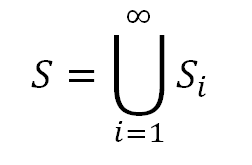where each Si is closed (this is just the definition of an Fσ set, i.e. a countable union of closed sets). We wish to define this set as an Fσ set in a slightly different way as a countable union of closed sets. We define a new series of sets Tj, where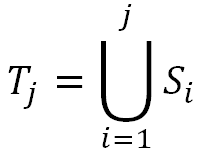In other words, T1 = S1, T2 = S1S2, and in general the Tj is the union of the first j sets of the collection of S-sets, {Si|iZ+}. Note that each Tj is a closed set, being a union of a finite number of closed sets (since j is finite), and that the union of all of the Tj is the same as the union of all of the Si, since each Tj is simply formed from a union of the Si. However, this new collection of sets possesses a useful feature in constructing our desired function f: each Tj is contained within the next member of the sequence Tj + 1.

Before defining the function itself, we need one more collection of sets based off of the Tj. These sets, for each j, essentially carry information about the differences between consecutive sets among the Tj. Symbolically, they are defined in the following way:What this means is that Uj contains those real numbers which are contained in the set difference of Tj and Tj - 1, or the set of numbers in Tj but not Tj - 1 (note in fact, that this set difference might be empty; we will return to this later). Note also that for j = 1, the definition of U1 makes use of a set "T0". We define this to be the empty set. Finally, among the real numbers contained in the set difference, only those which are on the boundary of the set (the condition preceding the "or" symbol, ∨) or are rational (the second condition, which states that such numbers are contained within the intersection of the set difference and the rational numbers, Q) are in Uj. The importance of these conditions will be made clear, but first we must finally define our long-sought function f: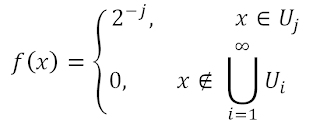Thus f takes a different constant value on each of the Uj, namely 2-j. This definition is well-defined because no two Uj intersect. If some member x of Um were a member of Un for n > m, then x would have to be contained in the set difference Tn - Tn - 1. However, since mn - 1, x must also be within Tn - 1, since the T's are nested. This is a contradiction, so the Uj are disjoint. Finally, the function f is defined as 0 outside of all of the Uj. Next, to illustrate how this definition works and is applied to sets, we consider a few examples.

Let S be the set {1/5,2,π}. To illustrate the concepts above, we initially assign S1 = {1/5}, S2 = {2}, S3 = {π}, and Sn = Ø for n ≥ 4 (note that this is not the only way to divide S into sets Si and that different divisions may yield different functions with the same discontinities). Clearly the union of the Si is indeed S. Next, T1 = S1 = {1/5}, T2 = S1S2 = {1/5,2}, T3 = S1S2S3 = {1/5,2,π}, and Tn = {1/5,2,π} for n ≥ 4, since the Si are empty above i = 3 and empty sets do not contribute to a union. Also, by definition, T0 is the empty set. Now we shall determine what form the Uj take. U1 takes elements from the set difference T1 - T0 = {1/5} - Ø = {1/5}. Since 1/5 is rational, it satisfies at least one of the additional conditions above and is indeed in U1. There are no other elements in T1 - T0, so U0 = {1/5}. Similarly, T2 - T1 contains one member, 2, and since it is rational, it is also the sole member of U2. Finally, T3 - T2 = {π}. Though π is not rational and so is not a member of (T3 - T2)∩Q, it still is contained within U3 because it is on the boundary of the set difference (the boundary of a set containing a single point is merely the same set). Thus U3 = {π}.

Using these facts, our previous definitions yield the following formula for f:This formula yields the graph below: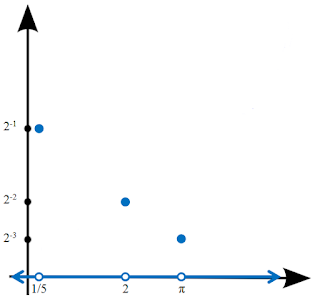Clearly this function has exactly our desired set S = {1/5,2,π} as its set of discontinuities. Note also the importance of the condition that those elements on the boundary of the set difference Ti - Ti - 1 are in Ui, as this was crucial in having π as a discontinuity, since it is irrational.

For a more intricate example, we now allow the set S to be the half-open interval (0,1]. S is Fσ because it is the union of the sets [1/2,1], [1/3,1/2], [1/4,1/3],..., each of which is closed. We therefore let Si = [1/(i + 1), 1/i] for every positive integer i. Clearly each number between 0 and 1 is contained in one of these sets, and 1 is contained in S1. However, the union of the infinite collection of sets is (0,1] because it does not include 0; if 0 were a member of the union, it would have to be a member of some Si. However, 0 is strictly less than 1/i for any positive integer i, and therefore cannot be in the union of the Si.

From these definitions for the Si, we see that T1 = S1 = [1/2,1], T2 = S1S2 = [1/3,1], and in general, Tj = [1/(j + 1),1]. Each set difference Tj - Tj - 1 is of the form [1/(j + 1),1/j), except for T1 - T0, which equals [1/2,1] and includes its right endpoint, since T0 is the empty set. As before, we select certain elements from each set difference to form the Uj, namely those which are rational (the boundary condition offers nothing new, since ∂(Tj - Tj - 1) = ∂[1/(j + 1),1/j) = {1/(j + 1)}, and 1/(j + 1) is rational). Thus each Uj contains exactly the the rationals between 1/(j + 1) and 1/j, including 1/(j + 1) but not including 1/j. Restricting our attention briefly to just one of these intervals, we see, for example in the vicinity of 2/3, which is in U1, that our function f will assume positive values at rationals and a value of zero at irrationals, since no irrational is a member of any Uj for this function. Our entire function will then consist of several smaller "copies" of the nowhere continuous Dirichlet function, which is 1 at rationals and 0 at irrationals. Before presenting a visualization of this function, we give its definition:The image below "graphs" this function. Due to the Dirichlet construction, the function jumps infinitely many times within every interval between 0 and 1. Therefore, when the function is split among the rationals and irrationals, the function's value at the rationals is given as a short-dashed line, and the function's value at the irrationals a long-dashed line. Note that at the value 2/3 below, the long-dashed line is at 0 and the short-dashed line at 2-1. Additionally, the segments on which the rationals are situated continued to shrink and move towards 0 as one approaches the origin, as indicated by the ellipsis.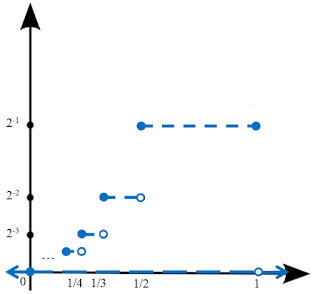It is easy to see that the function f is discontinuous on the entire interior of the unit interval, (0,1), because member of this set is surrounded arbitrarily closely by rational numbers, for which f is nonzero, and irrational numbers, for which f is zero. Additionally, since f(1) = 2-1, and f(x) = 0 for x > 1, f is additionally discontinuous at 1. However, to see that f is indeed discontinuous at exactly the set S, we must show the the function is not discontinuous, that is, continuous, at x = 0.

Consider the behavior of f(x) as x approaches 0. Given an arbitrarily small positive number ε, we can find a positive integer n such that 2-n < ε. As we know, on the interval [0,1/n), the largest value f can take is 2-n. Thus we have that for |x| < 1/n = δ, f(x) will be less than ε. Thus f is continuous at 0, and f has all the desired properties.

We have seen, for two examples, that the process above does yield a function f with discontinuities on exactly the members of a given Fσ set. After the above motivational examples, we finish the post by proving that this fact holds in the general case.

Let S be an Fσ set, f its corresponding function by the above scheme, and let the {Tj} be defined as above. We consider first the case of a point belonging to one of the set differences Tj - Tj - 1. If a point x is in the interior of this set difference f is discontinuous at x for the same reasons that the Dirichlet function is, since f behaves in the same manner as the Dirichlet function on the interiors of the set differences. If x is on the boundary of a set difference, f(x) = 2-j. However, neighboring points outside of the set difference must differ from this value by at least 2-(j + 1), since the closest possible value to 2-j that the function can take outside of Tj - Tj - 1 is 2-(j + 1), namely on the set difference Tj + 1 - Tj. Thus f is discontinuous at x. We have proven that f is discontinuous at every point of every set difference of the Tj. Since the union of the {Tj} is S, we see that S is exactly the union of all the set differences of the {Tj}. Thus f is discontinuous on S.

To finish the proof, we must show that f always continuous outside S. Let x now be any point outside of S, and therefore outside of every set difference. Since each Tj is closed, it contains its boundary, so x cannot be on the boundary of any Tj. Thus there is some neighborhood surrounding the point x that does not contain any member of Tj for some fixed value of j. For example, in the second example above, any set containing 0 in its interior contains part of a set Tj for some j, since the left endpoints of the {Tj} in that example, 1/(j + 1), become arbitrarily small. However, for any fixed Tj, say T3 = [1/4,1], we can choose a neighborhood of 0, for example (-1/5,1/5) which excludes all the {Tj} up to T3. This is the most difficult subtlety of the proof. Having found a neighborhood U of x (in the example, 0) that excludes the first n of the {Tj}, we note that the function must take values less than 2-n on U. Since this can be done for any finite n, we see that the function approaches 0 as one approaches x and that f is continuous at x. Therefore f is discontinuous on exactly the set S.

The above series describes a complete classification of discontinuities for real-valued functions. Also, this and related results give additional insight into the structure of real numbers, for example the distinction between rational numbers, on exactly which set a function can be discontinuous, and the irrational numbers, which are not Fσ. Such a classification demonstrates the power of mathematical techniques, as it provides simple conditions on all of the strange functions and sets which can exist on the real numbers.

Sources: Sources: Counterexamples in Analysis by Bernard R. Gelbaum and John M. H. Olmsted, Froda's Theorem on Wikipedia, http://holdenlee.wordpress.com/2010/04/26/can-a-function-be-continuous-only-on-rationals/, http://samjshah.com/2009/10/03/sin1x/,